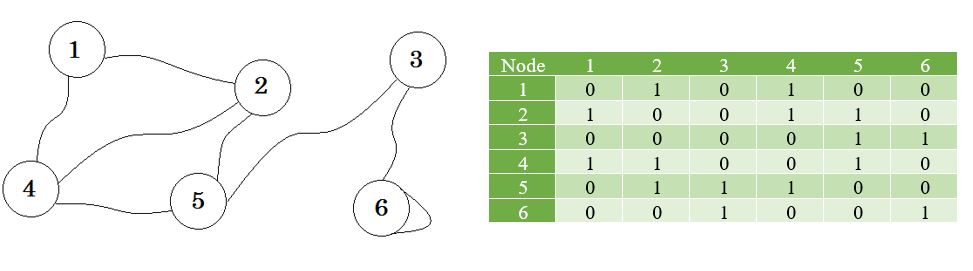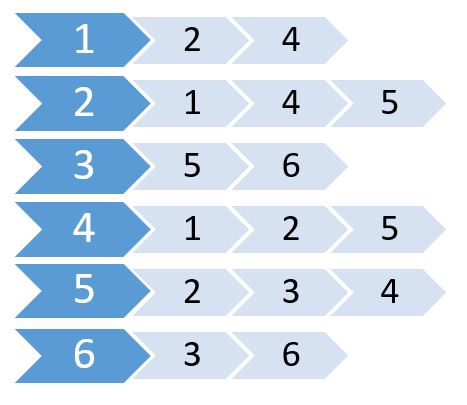suggest change

Adjacency list is a collection of unordered lists used to represent a finite graph. Each list describes the set of neighbors of a vertex in a graph. It takes less memory to store graphs.

Let’s see a graph, and its adjacency matrix:Now we create a list using these values.This is called adjacency list. It shows which nodes are connected to which nodes. We can store this information using a 2D array. But will cost us the same memory as Adjacency Matrix. Instead we are going to use dynamically allocated memory to store this one.

Many languages support Vector or List which we can use to store adjacency list. For these, we don’t need to specify the size of the List. We only need to specify the maximum number of nodes.

The pseudo-code will be:

``````Procedure Adjacency-List(maxN, E):       // maxN denotes the maximum number of nodes
edge[maxN] = Vector()                    // E denotes the number of edges
for i from 1 to E
input -> x, y                        // Here x, y denotes there is an edge between x, y
edge[x].push(y)
edge[y].push(x)
end for
Return edge``````

Since this one is an undirected graph, it there is an edge from x to y, there is also an edge from y to x. If it was a directed graph, we’d omit the second one. For weighted graphs, we need to store the cost too. We’ll create another vector or list named cost[] to store these. The pseudo-code:

``````Procedure Adjacency-List(maxN, E):
edge[maxN] = Vector()
cost[maxN] = Vector()
for i from 1 to E
input -> x, y, w
edge[x].push(y)
cost[x].push(w)
end for
Return edge, cost``````

From this one, we can easily find out the total number of nodes connected to any node, and what these nodes are. It takes less time than Adjacency Matrix. But if we needed to find out if there’s an edge between u and v, it’d have been easier if we kept an adjacency matrix.